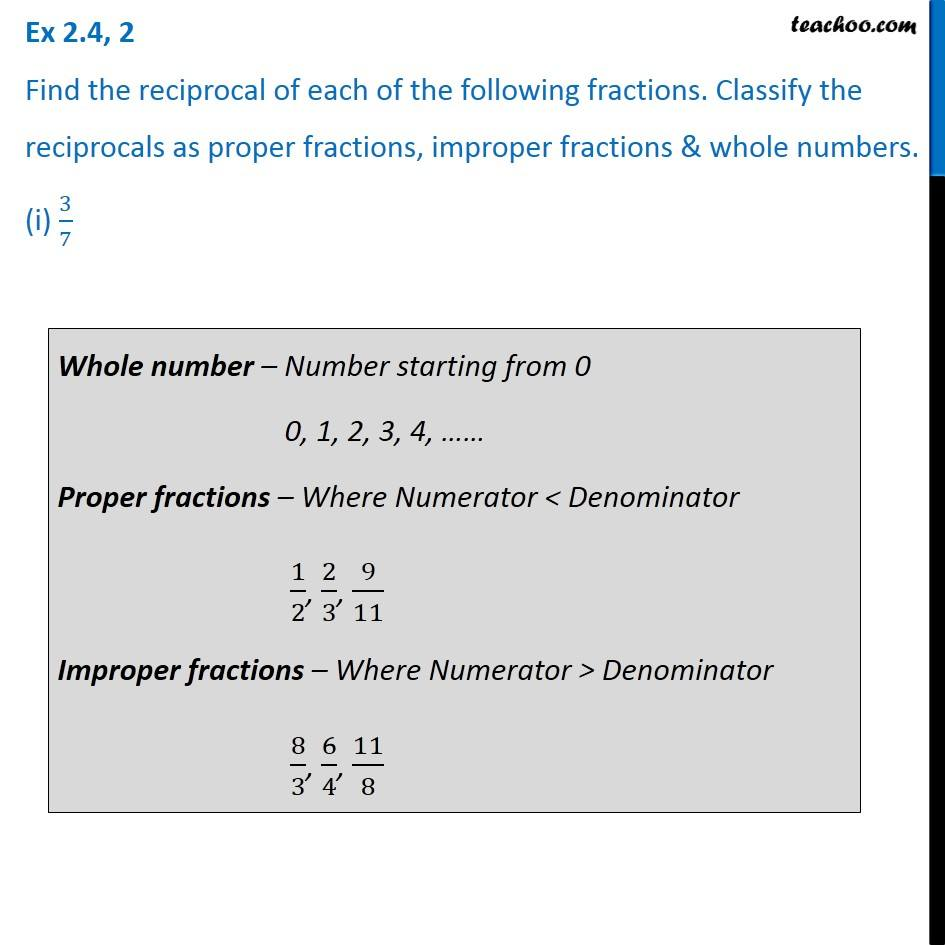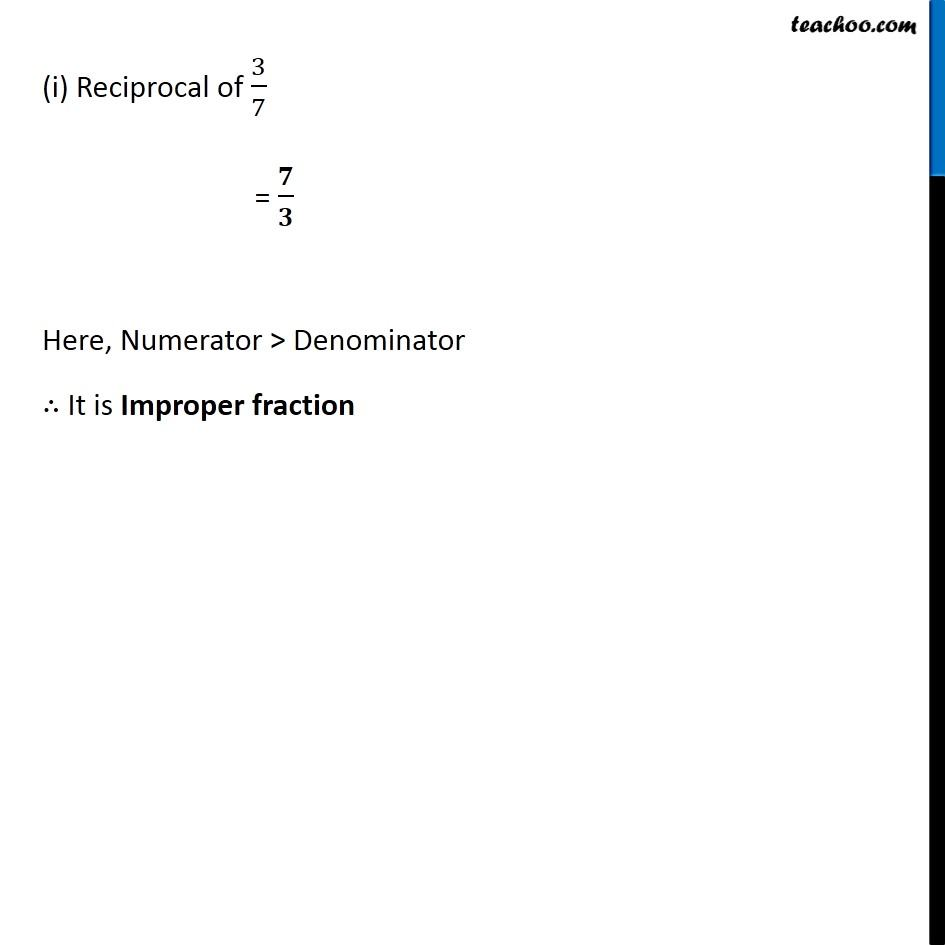Reciprocal of Fraction

Chapter 2 Class 7 Fractions and Decimals
Concept wiseIntroducing your new favourite teacher - Teachoo Black, at only ₹83 per month

### Transcript

Ex 2.4, 2 Find the reciprocal of each of the following fractions. Classify the reciprocals as proper fractions, improper fractions & whole numbers. (i) 3/7Whole number – Number starting from 0 0, 1, 2, 3, 4, …… Proper fractions – Where Numerator < Denominator 1/2, 2/3, 9/11 Improper fractions – Where Numerator > Denominator 8/3, 6/4, 11/8 (i) Reciprocal of 3/7 = 𝟕/𝟑 Here, Numerator > Denominator ∴ It is Improper fraction